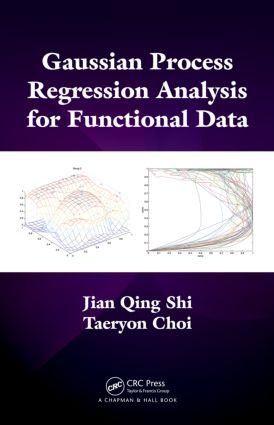# Gaussian Process Regression Analysis for Functional Data

## 1st Edition

Chapman and Hall/CRC

216 pages | 28 B/W Illus.

##### Purchasing Options:\$ = USD
Hardback: 9781439837733
pub: 2011-07-01
SAVE ~\$27.00
\$135.00
\$108.00
x
eBook (VitalSource) : 9780429151064
pub: 2011-07-01
from \$28.98

FREE Standard Shipping!

### Description

Gaussian Process Regression Analysis for Functional Data presents nonparametric statistical methods for functional regression analysis, specifically the methods based on a Gaussian process prior in a functional space. The authors focus on problems involving functional response variables and mixed covariates of functional and scalar variables.

Covering the basics of Gaussian process regression, the first several chapters discuss functional data analysis, theoretical aspects based on the asymptotic properties of Gaussian process regression models, and new methodological developments for high dimensional data and variable selection. The remainder of the text explores advanced topics of functional regression analysis, including novel nonparametric statistical methods for curve prediction, curve clustering, functional ANOVA, and functional regression analysis of batch data, repeated curves, and non-Gaussian data.

Many flexible models based on Gaussian processes provide efficient ways of model learning, interpreting model structure, and carrying out inference, particularly when dealing with large dimensional functional data. This book shows how to use these Gaussian process regression models in the analysis of functional data. Some MATLAB® and C codes are available on the first author’s website.

### Table of Contents

Introduction

Functional Regression Models

Gaussian Process Regression

Some Data Sets and Associated Statistical Problems

Bayesian Nonlinear Regression with Gaussian Process Priors

Gaussian Process Prior and Posterior

Posterior Consistency

Asymptotic Properties of the Gaussian Process Regression Models

Inference and Computation for Gaussian Process Regression Model

Empirical Bayes Estimates

Bayesian Inference and MCMC

Numerical Computation

Covariance Function and Model Selection

Examples of Covariance Functions

Selection of Covariance Functions

Variable Selection

Functional Regression Analysis

Linear Functional Regression Model

Gaussian Process Functional Regression Model

GPFR Model with a Linear Functional Mean Model

Mixed-Effects GPFR Models

GPFR ANOVA Model

Mixture Models and Curve Clustering

Mixture GPR Models

Mixtures of GPFR Models

Curve Clustering

Generalized Gaussian Process Regression for Non-Gaussian Functional Data

Gaussian Process Binary Regression Model

Generalized Gaussian Process Regression

Generalized GPFR Model for Batch Data

Mixture Models for Multinomial Batch Data

Some Other Related Models

Multivariate Gaussian Process Regression Model

Gaussian Process Latent Variable Models

Optimal Dynamic Control Using GPR Model

RKHS and Gaussian Process Regression

Appendices

Bibliography

Index

Further Reading and Notes appear at the end of each chapter.

### About the Authors

Jian Qing Shi, Ph.D., is a senior lecturer in statistics and the leader of the Applied Statistics and Probability Group at Newcastle University. He is a fellow of the Royal Statistical Society and associate editor of the Journal of the Royal Statistical Society (Series C). His research interests encompass functional data analysis using covariance kernel, incomplete data and model uncertainty, and covariance structural analysis and latent variable models.

Taeryon Choi, Ph.D., is an associate professor of statistics at Korea University. His research mainly focuses on the use of Bayesian methods and theory for various scientific problems.

### Subject Categories

##### BISAC Subject Codes/Headings:
MAT029000
MATHEMATICS / Probability & Statistics / General